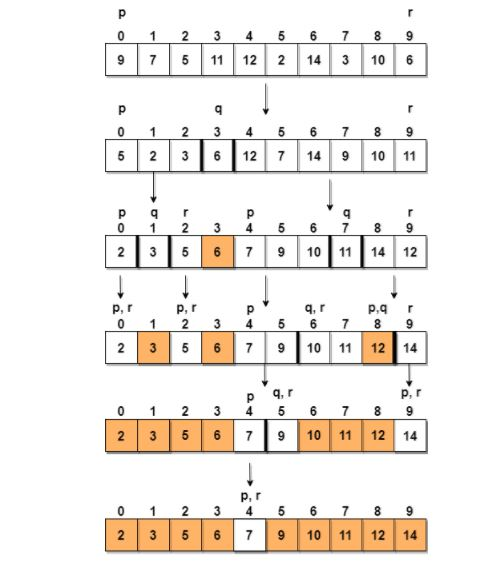# Chapter # 07 : Sorting and its Types

### Introduction to Sorting

Sorting is nothing but storage of data in sorted order, it can be in ascending or descending order. The term Sorting comes into the picture with the term Searching. There are so many things in our real life that we need to search, like a particular record in the database, roll numbers in the merit list, a particular telephone number, any particular page in a book etc.

Sorting arranges data in a sequence which makes searching easier. Every record which is going to be sorted will contain one key. Based on the key the record will be sorted. For example, suppose we have a record of students, every such record will have the following data:

• Roll No.

• Name

• Age

• Class

Here Student roll no. can be taken as a key for sorting the records in ascending or descending order. Now suppose we have to search a Student with roll no. 15, we don't need to search the complete record we will simply search for the Students with roll no. 10 to 20

### Types of Sorting Technique

There are many types of Sorting techniques, differentiated by their efficiency and space requirements. Following are some sorting techniques which we will be covering in next sections.

• Bubble Sort

• Insertion Sort

• Selection Sort

• Quick Sort

• Merge Sort

• Heap Sort

### Bubble Sorting

Bubble Sort is an algorithm which is used to sort N elements that are given in a memory for e.g. an Array with N number of elements. Bubble Sort compares all the element one by one and sort them based on their values.

It is called Bubble sort, because with each iteration the largest element in the list bubbles up towards the last place, just like a water bubble rises up to the water surface.

How Bubble Sort Works?

We take an unsorted array for our example.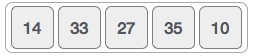Bubble sort starts with very first two elements, comparing them to check which one is greater.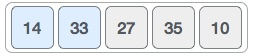In this case, value 33 is greater than 14, so it is already in sorted locations. Next, we compare 33 with 27.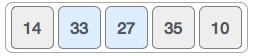We find that 27 is smaller than 33 and these two values must be swapped.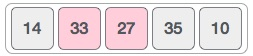The new array should look like this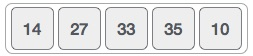Next we compare 33 and 35. We find that both are in already sorted positions.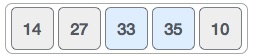Then we move to the next two values, 35 and 10.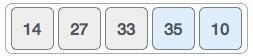We know then that 10 is smaller 35. Hence they are not sorted.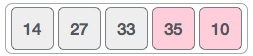We swap these values. We find that we have reached the end of the array. After one iteration, the array should look like this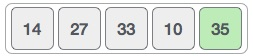To be precise, we are now showing how an array should look like after each iteration. After the second iteration, it should look like this.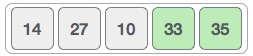Notice that after each iteration, at least one value moves at the end.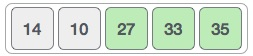And when there's no swap required, bubble sorts learns that an array is completely sorted.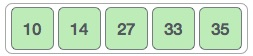## Algorithm

We assume list is an array of n elements. We further assume that swap function swaps the values of the given array elements.

begin BubbleSort(list)

​​ ​​​​ for all elements of list

​​ ​​ ​​ ​​ ​​​​ if list[i] > list[i+1]

​​ ​​ ​​ ​​ ​​ ​​ ​​ ​​​​ swap(list[i], list[i+1])

​​ ​​ ​​ ​​ ​​​​ end if

​​ ​​​​ end for

​​ ​​ ​​ ​​​​ return list  ​​​​

end BubbleSort

Procedure

procedure bubbleSort( list : array of items )

​​ ​​​​ loop = list.count;  ​​​​

​​ ​​​​ for i = 0 to loop-1 do:

​​ ​​ ​​ ​​ ​​​​ swapped = false

​​ ​​ ​​ ​​ ​​​​ for j = 0 to loop-1 do:  ​​ ​​ ​​ ​​​​

​​ ​​ ​​ ​​ ​​ ​​ ​​ ​​​​ /* compare the adjacent elements */  ​​​​

​​ ​​ ​​ ​​ ​​ ​​ ​​ ​​​​ if list[j] > list[j+1] then

​​ ​​ ​​ ​​ ​​ ​​ ​​ ​​ ​​ ​​ ​​​​ /* swap them */

​​ ​​ ​​ ​​ ​​ ​​ ​​ ​​ ​​ ​​ ​​​​ swap( list[j], list[j+1] )

​​ ​​ ​​ ​​ ​​ ​​ ​​ ​​ ​​ ​​ ​​​​ swapped = true

​​ ​​ ​​ ​​ ​​ ​​ ​​ ​​​​ end if  ​​ ​​ ​​ ​​ ​​ ​​ ​​​​

​​ ​​ ​​ ​​ ​​​​ end for  ​​ ​​ ​​ ​​​​

​​ ​​ ​​ ​​ ​​​​ /*if no number was swapped that means

​​ ​​ ​​ ​​ ​​​​ array is sorted now, break the loop.*/  ​​ ​​ ​​ ​​​​

​​ ​​ ​​ ​​ ​​​​ if(not swapped) then

​​ ​​ ​​ ​​ ​​ ​​ ​​ ​​​​ break

​​ ​​ ​​ ​​ ​​​​ end if  ​​ ​​ ​​​​

​​ ​​​​ end for  ​​​​

end procedure return list

### Insertion Sorting

It is a simple Sorting algorithm which sorts the array by shifting elements one by one. Following are some of the important characteristics of Insertion Sort.

• It has one of the simplest implementation

• It is efficient for smaller data sets, but very inefficient for larger lists.

• Insertion Sort is adaptive, that means it reduces its total number of steps if given a partially sorted list, hence it increases its efficiency.

• It is better than Selection Sort and Bubble Sort algorithms.

• Its space complexity is less. Like Bubble Sorting, insertion sort also requires a single additional memory space.

• It is a Stable sorting, as it does not change the relative order of elements with equal keys

How Insertion Sorting Works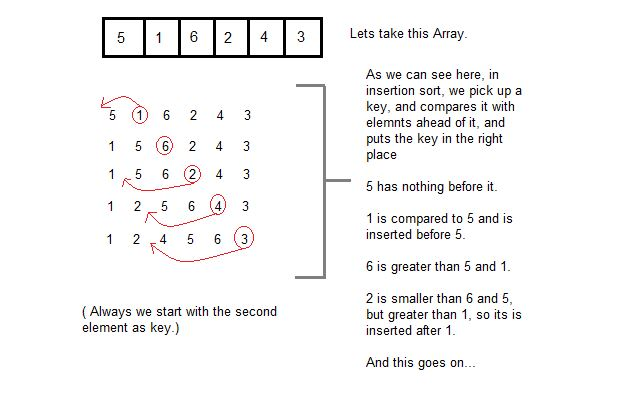### Algorithm

Now we have a bigger picture of how this sorting technique works, so we can derive simple steps by which we can achieve insertion sort.

Step 1 − If it is the first element, it is already sorted. return 1;

Step 2 − Pick next element

Step 3 − Compare with all elements in the sorted sub-list

Step 4 − Shift all the elements in the sorted sub-list that is greater than the

​​ ​​ ​​ ​​ ​​ ​​ ​​ ​​​​ value to be sorted

Step 5 − Insert the value

Step 6 − Repeat until list is sorted

Pseudocode

procedure insertionSort( A : array of items )

​​ ​​​​ int holePosition

​​ ​​​​ int valueToInsert

​​ ​​​​ for i = 1 to length(A) inclusive do:

​​ ​​ ​​ ​​ ​​​​ /* select value to be inserted */

​​ ​​ ​​ ​​ ​​​​ valueToInsert = A[i]

​​ ​​ ​​ ​​ ​​​​ holePosition = i  ​​ ​​ ​​ ​​​​

​​ ​​ ​​ ​​ ​​​​ /*locate hole position for the element to be inserted */

​​ ​​ ​​ ​​ ​​​​ while holePosition > 0 and A[holePosition-1] > valueToInsert do:

​​ ​​ ​​ ​​ ​​ ​​ ​​ ​​​​ A[holePosition] = A[holePosition-1]

​​ ​​ ​​ ​​ ​​ ​​ ​​ ​​​​ holePosition = holePosition -1

​​ ​​ ​​ ​​ ​​​​ end while

​​ ​​ ​​ ​​ ​​​​ /* insert the number at hole position */

​​ ​​ ​​ ​​ ​​​​ A[holePosition] = valueToInsert  ​​ ​​ ​​ ​​​​

​​ ​​​​ end for

end procedure

### Selection Sorting

Selection sort is a simple sorting algorithm. This sorting algorithm is an in-place comparison-based algorithm in which the list is divided into two parts, the sorted part at the left end and the unsorted part at the right end. Initially, the sorted part is empty and the unsorted part is the entire list.

The smallest element is selected from the unsorted array and swapped with the leftmost element, and that element becomes a part of the sorted array. This process continues moving unsorted array boundary by one element to the right.

How Selection Sort Works?

Consider the following array as an example.For the first position in the sorted list, the whole list is scanned sequentially. The first position where 14 is stored presently, we search the whole list and find that 10 is the lowest value.So we replace 14 with 10. After one iteration 10, which happens to be the minimum value in the list, appears in the first position of the sorted list.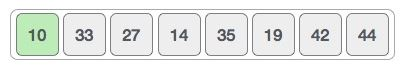For the second position, where 33 is residing, we start scanning the rest of the list in a linear manner.We find that 14 is the second lowest value in the list and it should appear at the second place. We swap these values.After two iterations, two least values are positioned at the beginning in a sorted manner.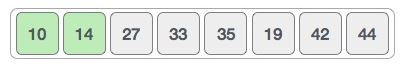The same process is applied to the rest of the items in the array.

Following is a pictorial depiction of the entire sorting process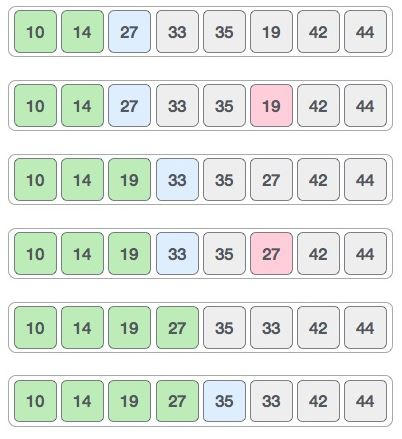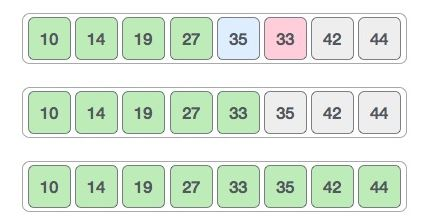Another Example

###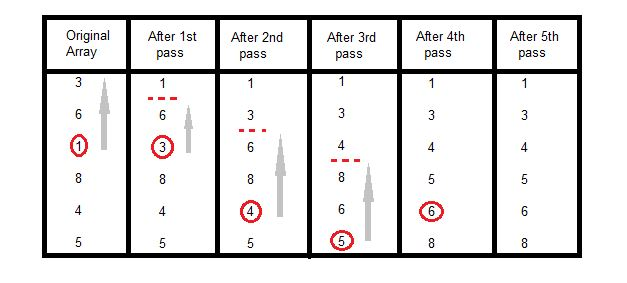In the first pass, the smallest element found is 1, so it is placed at the first position, then leaving the first element, next smallest element is searched from the rest of the elements. We get 3 as the smallest, so it is then placed at the second position. Then leaving 1 and 3, we search for the next smallest element from the rest of the elements and put it at third position and keep doing this until array is sorted.

### Algorithm

Step 1 − Set MIN to location 0

Step 2 − Search the minimum element in the list

Step 3 − Swap with value at location MIN

Step 4 − Increment MIN to point to next element

Step 5 − Repeat until list is sorted

### Pseudocode

procedure selection sort

​​ ​​​​ list ​​ : array of items

​​ ​​​​ n  ​​ ​​ ​​​​ : size of list

​​ ​​​​ for i = 1 to n - 1

​​ ​​​​ /* set current element as minimum*/

​​ ​​ ​​ ​​ ​​​​ min = i  ​​ ​​ ​​​​

​​ ​​ ​​ ​​ ​​​​ /* check the element to be minimum */

​​ ​​ ​​ ​​ ​​​​ for j = i+1 to n

​​ ​​ ​​ ​​ ​​ ​​ ​​ ​​​​ if list[j] < list[min] then

​​ ​​ ​​ ​​ ​​ ​​ ​​ ​​ ​​ ​​ ​​​​ min = j;

​​ ​​ ​​ ​​ ​​ ​​ ​​ ​​​​ end if

​​ ​​ ​​ ​​ ​​​​ end for

​​ ​​ ​​ ​​ ​​​​ /* swap the minimum element with the current element*/

​​ ​​ ​​ ​​ ​​​​ if indexMin != i ​​ then

​​ ​​ ​​ ​​ ​​ ​​ ​​ ​​​​ swap list[min] and list[i]

​​ ​​ ​​ ​​ ​​​​ end if

​​ ​​​​ end for

end procedure

### Merge Sorting

Merge sort is a sorting technique based on divide and conquer technique, Merge sort first divides the array into equal halves and then combines them in a sorted manner.

The concept of Divide and Conquer involves three steps:

• Divide the problem into multiple small problems.

• Conquer the sub problems by solving them. The idea is to break down the problem into atomic sub problems, where they are actually solved.

• Combine the solutions of the sub problems to find the solution of the actual problem.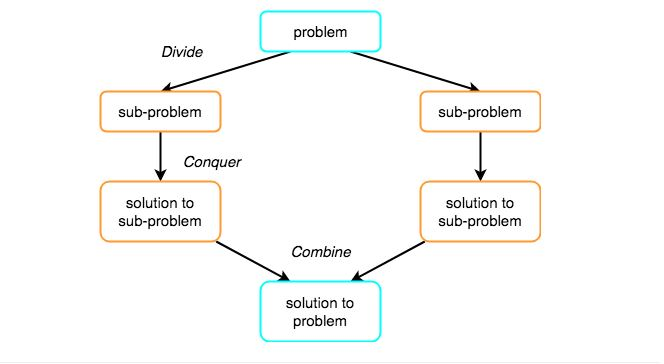As we have already discussed that merge sort utilizes divide-and-conquer rule to break the problem into sub-problems, the problem in this case being, sorting a given array.

In merge sort, we break the given array midway, for example if the original array had 6 elements, then merge sort will break it down into two subarrays with 3 elements each.

But breaking the original array into 2 smaller subarrays is not helping us in sorting the array.

So we will break these subarrays into even smaller subarrays, until we have multiple subarrays with single element in them. Now, the idea here is that an array with a single element is already sorted, so once we break the original array into subarrays which has only a single element, we have successfully broken down our problem into base problems.

And then we have to merge all these sorted subarrays, step by step to form one single sorted array.

Let's consider an array with values {14, 7, 3, 12, 9, 11, 6, 12}

Below, we have a pictorial representation of how merge sort will sort the given array.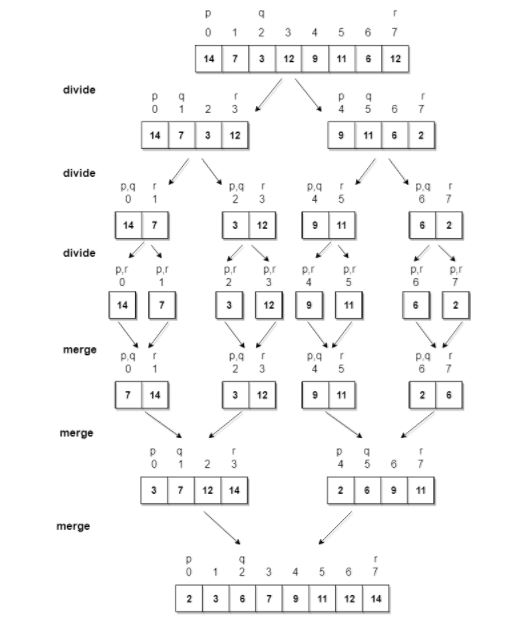In merge sort we follow the following steps:

• We take a variable p and store the starting index of our array in this. And we take another variable r and store the last index of array in it.

• Then we find the middle of the array using the formula (p + r)/2 and mark the middle index as q, and break the array into two subarrays, from p to q and from q + 1 to r index.

• Then we divide these 2 subarrays again, just like we divided our main array and this continues.

• Once we have divided the main array into subarrays with single elements, then we start merging the subarrays.

### Quick Sorting

Quick Sort Pivot Algorithm

Based on our understanding of partitioning in quick sort, we will now try to write an algorithm for it, which is as follows.

Step 1 − Choose the highest index value has pivot

Step 2 − Take two variables to point left and right of the list excluding pivot

Step 3 − left points to the low index

Step 4 − right points to the high

Step 5 − while value at left is less than pivot move right

Step 6 − while value at right is greater than pivot move left

Step 7 − if both step 5 and step 6 does not match swap left and right

Step 8 − if left ≥ right, the point where they met is new pivot

• After selecting an element as pivot, which is the last index of the array in our case, we divide the array for the first time.

• In quick sort, we call this partitioning. It is not simple breaking down of array into 2 subarrays, but in case of partitioning, the array elements are so positioned that all the elements smaller than the pivot will be on the left side of the pivot and all the elements greater than the pivot will be on the right side of it.

• And the pivot element will be at its final sorted position.

• The elements to the left and right, may not be sorted.

• Then we pick subarrays, elements on the left of pivot and elements on the right of pivot, and we perform partitioning on them by choosing a pivot in the subarrays.

Let's consider an array with values {9, 7, 5, 11, 12, 2, 14, 3, 10, 6}

Below, we have a pictorial representation of how quick sort will sort the given array.